# The PDLREG Procedure

## Overview: PDLREG Procedure

The PDLREG procedure estimates regression models for time series data in which the effects of some of the regressor variables are distributed across time. The distributed lag model assumes that the effect of an input variable X on an output Y is distributed over time. If you change the value of X at time t, Y will experience some immediate effect at time t, and it will also experience a delayed effect at times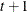,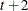, and so on up to time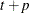for some limit p.

The regression model supported by PROC PDLREG can include any number of regressors with distribution lags and any number of covariates. (Simple regressors without lag distributions are called covariates.) For example, the two-regressor model with a distributed lag effect for one regressor is written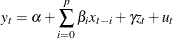Here,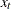is the regressor with a distributed lag effect,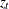is a simple covariate, and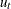is an error term.

The distribution of the lagged effects is modeled by Almon lag polynomials. The coefficients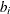of the lagged values of the regressor are assumed to lie on a polynomial curve. That is,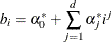where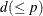is the degree of the polynomial. For the numerically efficient estimation, the PDLREG procedure uses orthogonal polynomials. The preceding equation can be transformed into orthogonal polynomials: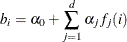where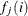is a polynomial of degree j in the lag length i, and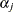is a coefficient estimated from the data.

The PDLREG procedure supports endpoint restrictions for the polynomial. That is, you can constrain the estimated polynomial lag distribution curve so that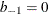or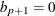, or both. You can also impose linear restrictions on the parameter estimates for the covariates.

You can specify a minimum degree and a maximum degree for the lag distribution polynomial, and the procedure fits polynomials for all degrees in the specified range. (However, if distributed lags are specified for more that one regressor, you can specify a range of degrees for only one of them.)

The PDLREG procedure can also test for autocorrelated residuals and perform autocorrelated error correction by using the autoregressive error model. You can specify any order autoregressive error model and can specify several different estimation methods for the autoregressive model, including exact maximum likelihood.

The PDLREG procedure computes generalized Durbin-Watson statistics to test for autocorrelated residuals. For models with lagged dependent variables, the procedure can produce Durbin h and Durbin t statistics. You can request significance level p-values for the Durbin-Watson, Durbin h, and Durbin t statistics. See Chapter 8: The AUTOREG Procedure, for details about these statistics.

The PDLREG procedure assumes that the input observations form a time series. Thus, the PDLREG procedure should be used only for ordered and equally spaced time series data.